# Day Number of Year, Power Query Custom Function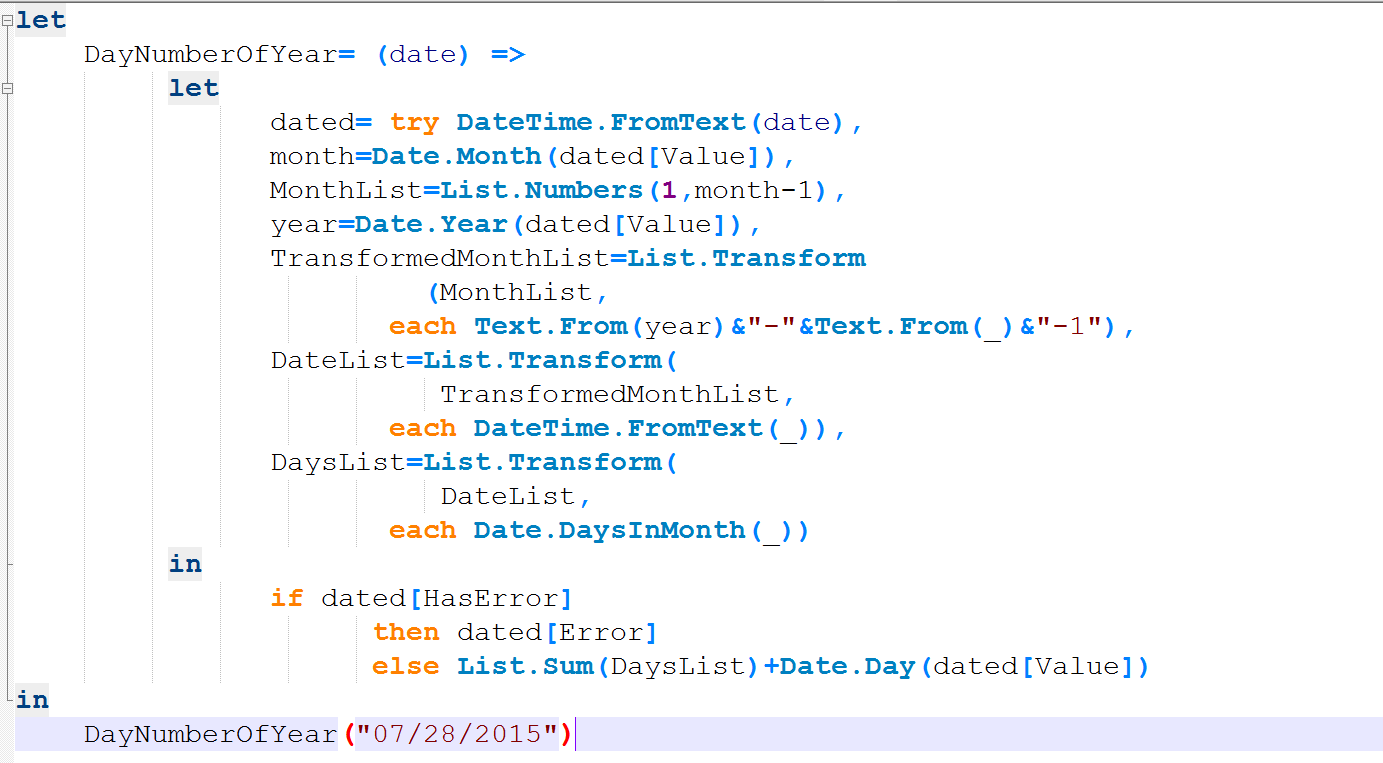There are number of Date and DateTime built-in functions in Power Query which are really helpful. There is also a function for DayNumberOfYear. However I’ve thought it would be a good example to go through writing a function that uses Generators, Each singleton function, and error handling all inside a custom function. Through this post you will also learn;

• how to create Custom Function
• how to use Generators as loop structure
• and how to user Error Handling.

Let’s consider this date as today’s date : 28th of July of 2015 (this is actually the date of this blog post)

There might be number of methods to calculate the day number of year for this date (which is 209). I just use one of them here. Steps are as below;

• fetch number of days for each month from January of this year (of the date above) to previous month (of the date above).
• Calculate sum of values above will give me the number of days in all month prior than this month.
• add the day number of the date to the calculated sum above.

Some of calculations can be helped through with Power Query Date functions. So let’s start;

1 – Create a function in Power Query called DayNumberOfYear as below

If you don’t know where to write below code:

• Open Excel, Go to Power Query Tab, Click On Get Data from Source, Blank Query, In the Query Editor window go to View tab, and click on Advanced Editor.
• Open Power BI, click on Edit Queries, In the Query Editor window go to View tab, and click on Advanced Editor.
```let                                          //start of the code
DayNumberOfYear= (date) =>                //Function name, and input parameter
let                                  //start of function body
dated=DateTime.FromText(date)  //date conversion from text
in                                   //start of function output
dated                          //function body output
in                                            //start of output lines generated
DayNumberOfYear("07/28/2015")             //call function by a value```

I’ve put some comments in above script to help you understand each line. In general DayNumberOfYear is name of the function. It accepts an input parameter “date”. and convert the parameter from text value to DateTime. the last line of the code calls the function with specific date (“07/28/2015”).

** Note that Date Conversion function is locale dependent. So if the date time of your system is no MM/DD/YYYY then you have to enter date as it formatted in your system (look below the clock on right hand side bottom of your monitor to check the format).

the result of above script will be:

07/28/2015 12:00:00 a.m.

2 – Fetch Month Number and generate a list of all prior months.

Fetching month number is easily possible with Date.Month function. the remaining part is looping through months from January of this year to previous month (of the given date). Unfortunately there is no loop structure in Power Query M language yet, but fortunately we can use Generator functions for that. Generator function is a function that produce/generate a list based on some parameters. For example you can generate a list of dates from a start date, based on given occurrence of a period of time. or you can generate a list of numbers. For this example we want to generate list of numbers, starting from 1 (month January) to the current month number minus 1 (previous month).

Here is the code:

```let
DayNumberOfYear= (date) =>
let
dated=DateTime.FromText(date),
month=Date.Month(dated),//month number
MonthList=List.Numbers(1,month-1) // generate list of months from Jan to previous month
in
MonthList
in
DayNumberOfYear("07/28/2015")```

The result is a list of month numbers as below:Generator Function used in above code is List.Numbers. this function generate list of numbers starting from a value.

3 – Transform list to full date list

We have to calculate number of days for each month in the function. Number of days in each month can be fetched by Date.DaysInMonth function. However this function accept a full DateTime data type, and the value that we have in our list members are text. so we have to produce a datetime value from it. for generating a full date we need the year portion as well, we use Date.Year function to fetch that.

Here is the code to transform the list:

```let
DayNumberOfYear= (date) =>
let
dated=DateTime.FromText(date),
month=Date.Month(dated),
MonthList=List.Numbers(1,month-1),
year=Date.Year(dated), // fetch year
TransformedMonthList=List.Transform  // transform list
(MonthList,
each Text.From(year)&"-"&Text.From(_)&"-1") // generate full text value
in
TransformedMonthList
in
DayNumberOfYear("07/28/2015")```

The result is: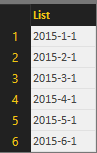As you see the above result is not still of datetime data type, we’ve only generated full date as text value. Now we can convert values to datetime data type

Here is the code:

```let
DayNumberOfYear= (date) =>
let
dated=DateTime.FromText(date),
month=Date.Month(dated),
MonthList=List.Numbers(1,month-1),
year=Date.Year(dated),
TransformedMonthList=List.Transform
(MonthList,
each Text.From(year)&"-"&Text.From(_)&"-1"),
DateList=List.Transform(
TransformedMonthList,
each DateTime.FromText(_))//transform to DateTime value
in
DateList
in
DayNumberOfYear("07/28/2015")```

and the result: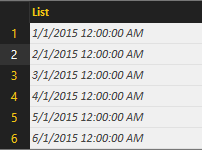4 – Transform the list to List of DaysNumberOfMonths

We use DaysInMonth function to fetch number of days in each month from the list. here is the code:

```let
DayNumberOfYear= (date) =>
let
dated=DateTime.FromText(date),
month=Date.Month(dated),
MonthList=List.Numbers(1,month-1),
year=Date.Year(dated),
TransformedMonthList=List.Transform
(MonthList,
each Text.From(year)&"-"&Text.From(_)&"-1"),
DateList=List.Transform(
TransformedMonthList,
each DateTime.FromText(_)),
DaysList=List.Transform(
DateList,
each Date.DaysInMonth(_))
in
DaysList
in
DayNumberOfYear("07/28/2015")```

and the result:5 – Calculate Sum of Dates and Add day number of this month to it

The list is ready to use, we need to sum it up only. And then add the current day Date.Day from the given date to it.

Here is the code:

```let
DayNumberOfYear= (date) =>
let
dated=DateTime.FromText(date),
month=Date.Month(dated),
MonthList=List.Numbers(1,month-1),
year=Date.Year(dated),
TransformedMonthList=List.Transform
(MonthList,
each Text.From(year)&"-"&Text.From(_)&"-1"),
DateList=List.Transform(
TransformedMonthList,
each DateTime.FromText(_)),
DaysList=List.Transform(
DateList,
each Date.DaysInMonth(_))
in
List.Sum(DaysList)//sum of values in the list
+Date.Day(dated)//current date's day number
in
DayNumberOfYear("07/28/2015")```

and the result is:6- Error Handling

The function is working, but if the given date format be wrong the we will face and error such as below: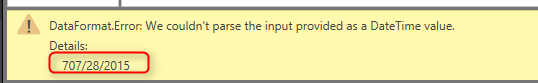So let’s add few lines of error handling to the code. We can simply use Try clause to the code as below:

```let
DayNumberOfYear= (date) =>
let
dated= try DateTime.FromText(date),
month=Date.Month(dated[Value]),
MonthList=List.Numbers(1,month-1),
year=Date.Year(dated[Value]),
TransformedMonthList=List.Transform
(MonthList,
each Text.From(year)&"-"&Text.From(_)&"-1"),
DateList=List.Transform(
TransformedMonthList,
each DateTime.FromText(_)),
DaysList=List.Transform(
DateList,
each Date.DaysInMonth(_))
in
if dated[HasError]
then dated[Error]
else List.Sum(DaysList)+Date.Day(dated[Value])
in
DayNumberOfYear("707/28/2015")```

Result for bad formatted given date is :Here is the full code of the script:

```let
DayNumberOfYear= (date) =>
let
dated= try DateTime.FromText(date),
month=Date.Month(dated[Value]),
MonthList=List.Numbers(1,month-1),
year=Date.Year(dated[Value]),
TransformedMonthList=List.Transform
(MonthList,
each Text.From(year)&"-"&Text.From(_)&"-1"),
DateList=List.Transform(
TransformedMonthList,
each DateTime.FromText(_)),
DaysList=List.Transform(
DateList,
each Date.DaysInMonth(_))
in
if dated[HasError]
then dated[Error]
else List.Sum(DaysList)+Date.Day(dated[Value])
in
DayNumberOfYear("707/28/2015")```

In this post you’ve learned:

• A Function that calculate Day Number of Year for a given date
• Creating Custom Function
• using Generators as Loop structure
• Error Handling

Save

Trainer, Consultant, Mentor
Reza Rad is a Microsoft Regional Director, an Author, Trainer, Speaker and Consultant. He has a BSc in Computer engineering; he has more than 20 years’ experience in data analysis, BI, databases, programming, and development mostly on Microsoft technologies. He is a Microsoft Data Platform MVP for 12 continuous years (from 2011 till now) for his dedication in Microsoft BI. Reza is an active blogger and co-founder of RADACAD. Reza is also co-founder and co-organizer of Difinity conference in New Zealand, Power BI Summit, and Data Insight Summit.
Reza is author of more than 14 books on Microsoft Business Intelligence, most of these books are published under Power BI category. Among these are books such as Power BI DAX Simplified, Pro Power BI Architecture, Power BI from Rookie to Rock Star, Power Query books series, Row-Level Security in Power BI and etc.
He is an International Speaker in Microsoft Ignite, Microsoft Business Applications Summit, Data Insight Summit, PASS Summit, SQL Saturday and SQL user groups. And He is a Microsoft Certified Trainer.
Reza’s passion is to help you find the best data solution, he is Data enthusiast.
His articles on different aspects of technologies, especially on MS BI, can be found on his blog: https://radacad.com/blog.

## 3 thoughts on “Day Number of Year, Power Query Custom Function”

•Henrik says:

Hi,

Thanks for a very good tutorial on building functions! Great work!

Regards

Henrik from Sweden

•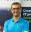Reza Rad says:

Hi Henrik,